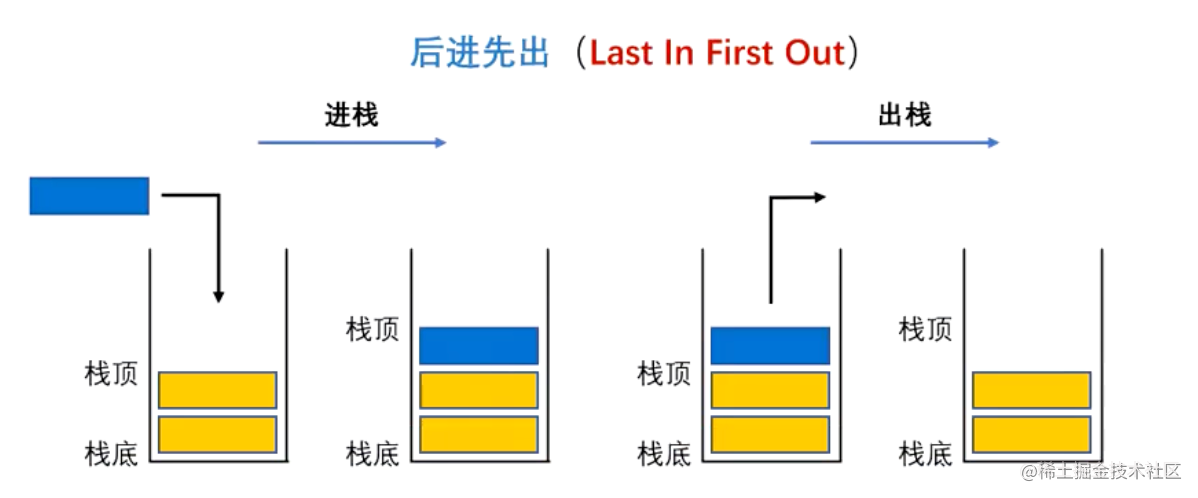2023-02-0100

2.1 基于数组 v1 版
2.2 使用泛型重构 v2 版
3. 实战一：有效的括号
3.1 题目描述
3.2 题目分析
3.3 解一：栈
4. 实战二：下一个更大元素 I
4.1 题目描述
4.2 解一：暴力
4.3 解二：单调栈

# 1. 认识栈结构

1. 栈是一种 后进先出（LIFO） 的数据结构

2. `js` 中没有栈，但我们可以用 数组或链表 实现栈的所有功能

3. 栈的常用操作:

1. `push（入栈）`
2. `pop（出栈）`
3. `peek（返回栈顶元素）`
4. `isEmpty（判断是否为空栈）`
5. `size（返回栈里元素个数）`# 2. 实现栈结构的封装

1. 基于 数组 实现
2. 基于 链表 实现

## 2.1 基于数组 v1 版

```typescript```// 封装一个栈
class ArrayStack {
// 定义一个数组，用于存储元素
private data: any[] = [];

// 实现栈中相关的操作方法
// push 方法：将一个元素压入栈中
push(element: any): void {
this.data.push(element);
}

// pop方法：将栈顶的元素弹出栈（返回出去，并且从栈顶移除）
pop(): any {
return this.data.pop();
}

// peek方法：返回栈顶元素
peek(): any {
return this.data[this.data.length - 1];
}

// isEmpty方法：判断栈是否为空
isEmpty(): boolean {
return this.data.length === 0;
}

// size放法：返回栈里元素个数
size(): number {
return this.data.length;
}
}
``````

```typescript```const as = new ArrayStack();
as.push(1);
as.push(2);
as.pop();
as.push(3);
console.log(as); // ArrayStack { data: [ 1, 3 ] }
``````

## 2.2 使用泛型重构 v2 版

```ts```// 封装一个栈
class ArrayStack<T = any> {
// 定义一个数组，用于存储元素
private data: T[] = [];

// 实现栈中相关的操作方法
// push 方法：将一个元素压入栈中
push(element: T): void {
this.data.push(element);
}

// pop方法：将栈顶的元素弹出栈（返回出去，并且从栈顶移除）
pop(): T | undefined {
return this.data.pop();
}

// peek方法：返回栈顶元素
peek(): T | undefined {
return this.data[this.data.length - 1];
}

// isEmpty方法：判断栈是否为空
isEmpty(): boolean {
return this.data.length === 0;
}

// size放法：返回栈里元素个数
size(): number {
return this.data.length;
}
}
``````

```ts```const as = new ArrayStack<number>();
as.push(1);
as.push('2'); // ✖️ 类型“string”的参数不能赋给类型“number”的参数。
as.push(2);
as.pop();
as.push(3);
console.log(as);
``````

# 3. 实战一：有效的括号

## 3.1 题目描述

1. 左括号必须用相同类型的右括号闭合。
2. 左括号必须以正确的顺序闭合。
3. 每个右括号都有一个对应的相同类型的左括号。

``````输入： s = "()"

``````

``````输入： s = "()[]{}"

``````

``````输入： s = "(]"

``````

• `1 <= s.length <= 104`
• `s` 仅由括号 `'()[]{}'` 组成

## 3.2 题目分析

1. 我们只需要维护一个栈结构
2. 遍历给定的字符串 `s`
1. 遇到 `[``{``(` 这三种符号时将它们压入栈，
2. 遇到 `]``}``)` 这三种符号时就取出栈顶元素与之对比，如果不能够组成有效括号则函数直接返回 `false`，如果能则进入下个循环比较
3. 知道循环结束，判断栈中元素如果为空则表示字符串有效，反之则无效

## 3.3 解一：栈

```ts```function isValid(s: string): boolean {
const stack = new ArrayStack<string>();

for (let i = 0; i < s.length; i++) {
const c = s[i];
switch (c) {
case "{":
stack.push("}");
break;
case "[":
stack.push("]");
break;
case "(":
stack.push(")");
break;
default:
if (stack.pop() !== c) return false;
}
}
return stack.isEmpty();
}
``````

# 4. 实战二：下一个更大元素 I

## 4.1 题目描述

`nums1` 中数字 `x` 的 下一个更大元素 是指 `x` 在 `nums2` 中对应位置 右侧 的 第一个 比 `x` ****大的元素。

``````输入： nums1 = [4,1,2], nums2 = [1,3,4,2].

- 4 ，用加粗斜体标识，nums2 = [1,3,4,2]。不存在下一个更大元素，所以答案是 -1 。
- 1 ，用加粗斜体标识，nums2 = [1,3,4,2]。下一个更大元素是 3 。
- 2 ，用加粗斜体标识，nums2 = [1,3,4,2]。不存在下一个更大元素，所以答案是 -1 。
``````

``````输入： nums1 = [2,4], nums2 = [1,2,3,4].

- 2 ，用加粗斜体标识，nums2 = [1,2,3,4]。下一个更大元素是 3 。
- 4 ，用加粗斜体标识，nums2 = [1,2,3,4]。不存在下一个更大元素，所以答案是 -1 。
``````

• `1 <= nums1.length <= nums2.length <= 1000`
• `0 <= nums1[i], nums2[i] <= 104`
• `nums1``nums2`中所有整数 互不相同
• `nums1` 中的所有整数同样出现在 `nums2` 中

## 4.2 解一：暴力

1. 双重循环遍历 `nums1``nums2` 两个数组
2. 在第一层遍历 `nums1` 循环中，找出 `nums1[i]` 对应 在 `nums2` 中的下标位置 `pos`
3. `pos + 1` 位置开始遍历 nums2 数组，查找比 `nums[i]` 大的数字

```ts```function nextGreaterElement(nums1: number[], nums2: number[]): number[] {
let res: number[] = [];
for (let i = 0; i < nums1.length; i++) {
let pos: number = 0;
for (let j = 0; j < nums2.length; j++) {
if (nums2[j] === nums1[i]) {
pos = j;
break;
}
}
if (pos === nums2.length - 1) res.push(-1);

for (let j = pos + 1; j < nums2.length; j++) {
if (nums2[j] > nums1[i]) {
res.push(nums2[j]);
break;
}
if (j >= nums2.length - 1) res.push(-1);
}
}
return res;
}
``````

• 时间复杂度：`O(mn)` ，其中 `m``nums1` 的长度，`n``nums2` 的长度。

• 空间复杂度：`O(1)`

## 4.3 解二：单调栈

1. 创建一个 `map`（哈希表），它的 `key``nums2` 中的值，`value``key` 值右侧的 下一个更大元素
2. 维护一个 `stack` 单调栈，倒序遍历 `nums2` 数组
3. 在循环中比较 `nums2[i]` 与 单调栈中的值，将小于 `nums2[i]` 的值 `pop` 出，最后剩下的都是比 `nums2[i]` 大的数，且栈顶的值就是下一个更大元素
4. 使用 `map` 哈希表记录每个 `nums2[i]` 对应目标值。
```ts```function nextGreaterElement(nums1: number[], nums2: number[]): number[] {
const map = new Map<number, number>();
const stack = new ArrayStack<number>();
for (let i = nums2.length - 1; i >= 0; --i) {
const num = nums2[i];
while (stack.size() && num >= (stack.peek() as number)) {
stack.pop();
}
map.set(num, stack.size() ? (stack.peek() as number) : -1);
stack.push(num);
}
const res = new Array(nums1.length).fill(0).map((_, i) => map.get(nums1[i]) as number);
return res;
}
``````

• 时间复杂度：`O(m + n)` ，其中 `m``nums1` 的长度，`n``nums2` 的长度。

• 空间复杂度：`O(n)` 用于存储哈希表 `map`